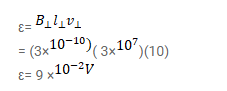# A 10 m wide spacecraft moves through the interstellar space at

Question:

A $10 \mathrm{~m}$ wide spacecraft moves through the interstellar space at a speed $3 \times 10^{7} \mathrm{~m} / \mathrm{s}$. A magnetic field $B=3 \times 10^{-10} \mathrm{~T}$ exists in the space in a direction perpendicular to the plane of motion. Treating the spacecraft as a conductor, calculate the emf induced across ita width.

Solution: